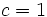Lazard Lie group

This article defines a group property: a property that can be evaluated to true/false for any given group, invariant under isomorphism
View a complete list of group properties
VIEW RELATED: Group property implications | Group property non-implications |Group metaproperty satisfactions | Group metaproperty dissatisfactions | Group property satisfactions | Group property dissatisfactions

BEWARE! This term is nonstandard and is being used locally within the wiki. [SHOW MORE]

Definition

Quick definition

A group is termed a Lazard Lie group if its 3-local nilpotency class is finite and less than or equal to the group's powering threshold.

Explicit definition

A group$G$ is termed a class$c$ Lazard Lie group for some natural number$c$ if both the following hold:

No. Shorthand for property Explanation
1 The powering threshold for$G$ is at least$c$, i.e.,$G$ is powered for the set of all primes less than or equal to$c$.$G$ is uniquely$p$-divisible for all primes$p \le c$. In other words, if$p \le c$ is a prime and$g \in G$, there is a unique value$h \in G$ satisfying$h^p = g$.
2 The 3-local nilpotency class of$G$ is at most$c$. For any three elements of$G$, the subgroup of$G$ generated by these three elements is a nilpotent group of nilpotency class at most$c$.

Condition (1) gets more demanding (i.e., stronger, so satisfied by fewer groups) as$c$ increases, while condition (2) gets less demanding (i.e., weaker, so satisfied by more groups) as we increase$c$. Thus, a particular value of$c$ may work for a group but larger and smaller values may not.

A group is termed a Lazard Lie group if it is a class$c$ Lazard Lie group for some natural number$c$.

A Lazard Lie group is a group that can participate on the group side of the Lazard correspondence. The Lie ring on the other side is its Lazard Lie ring.

Set of possible values$c$ for which a group is a class$c$ Lazard Lie group

A group is a Lazard Lie group if and only if its 3-local nilpotency class is less than or equal to its powering threshold. The set of permissible$c$ values for which the group is a class$c$ Lazard Lie group is the set of$c$ satisfying:

3-local nilpotency class$\le c \le$ powering threshold

p-group version

A p-group is termed a Lazard Lie group if its 3-local nilpotency class is at most$p - 1$. In other words, every subgroup of it generated by at most three elements has nilpotency class at most$p - 1$ where$p$ is the prime associated with the group.

Metaproperties

Metaproperty name Satisfied? Proof Statement with symbols
subgroup-closed group property No Lazard Lie property is not subgroup-closed It is possible to have a Lazard Lie group$G$ and a subgroup$H$ of$G$ such that$H$ is not a Lazard Lie group in its own right.
quotient-closed group property No Lazard Lie property is not quotient-closed It is possible to have a Lazard Lie group$G$ and a normal subgroup$H$ of$G$ such that the quotient group$G/H$ is not a Lazard Lie group in its own right.
finite direct product-closed group property No Lazard Lie property is not finite direct product-closed It is possible to have Lazard Lie groups$G_1$ and$G_2$ such that the external direct product$G_1 \times G_2$ is not a Lazard Lie group.

Relation with other properties

Stronger properties

Property Meaning Proof of implication Proof of strictness (reverse implication failure) Intermediate notions
abelian group any two elements commute Precisely the case$c = 1$ (see also Lazard correspondence#Particular cases) |FULL LIST, MORE INFO
Baer Lie group uniquely 2-divisible and class at most two Precisely the case$c = 2$ (see also Lazard correspondence#Particular cases Global Lazard Lie group|FULL LIST, MORE INFO
p-group of nilpotency class less than p global nilpotency class puts an upper bound on the 3-local nilpotency class Global Lazard Lie group|FULL LIST, MORE INFO
rationally powered nilpotent group nilpotent and uniquely divisible for all primes Global Lazard Lie group|FULL LIST, MORE INFO